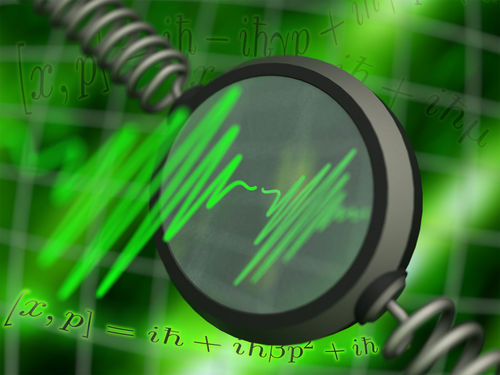19.03.2012

Looking at quantum gravity in a mirror

Einstein’s theory of gravity and quantum physics are expected to merge at the Planck-scale of extremely high energies and on very short distances. At this scale, new phenomena could arise. However, the Planck-scale is so remote from current experimental capabilities that tests of quantum gravity are widely believed to be nearly impossible. Now an international collaboration between the groups of Prof. C. Brukner and Prof. M. Aspelmeyer at the University of Vienna and Prof. M. S. Kim at Imperial College London has proposed a new quantum experiment using Planck-mass mirrors. Such an experiment could test certain predictions made by quantum gravity proposals in the laboratory. The findings will be published this week in “Nature Physics”.A long-standing challenge

The search for a theory that unifies quantum mechanics with Einstein's theory of gravity is one of the main challenges in modern physics. Quantum mechanics describes effects at the scale of single particles, atoms and molecules. Einstein's theory of gravity, on the other hand, is typically relevant for large masses. It is widely expected that phenomena stemming from a unified theory of quantum gravity will become evident only at the so-called Planck-scale of extremely high energies or extremely small distances. The Planck-length is 1.6 x 10-35 meters: This is so small that if one were to take this scale to be 1 meter, then an atom would be as large as the entire visible Universe! Similarly, the Planck-energy is so large that even the Large Hadron Collider in CERN only reaches an insignificantly tiny fraction of this energy, and a particle accelerator would need to be of astronomical size to get even close to the Planck-Energy. This scale is also described by the Planck-mass: A piece of dust weights about that much, which is truly heavy compared to single atoms, and quantum phenomena are typically considered unobservable for such masses. The Planck-scale is therefore so remote from current experimental capabilities that tests of quantum gravity proposals are widely believed to be nearly impossible. However, physicists have now found a way to probe some predictions of quantum gravity proposals in the laboratory by looking at quantum effects in Planck-mass quantum systems.

The sequence makes the difference

In quantum mechanics it is impossible to know where a particle is and how fast it is moving at the same time. Nevertheless, it is possible to make two subsequent measurements: a measurement of the particle's position followed by a measurement of its momentum, or vice-versa. In quantum physics the two different measurement sequences produces different experimental results. According to many theories of quantum gravity, this difference would be altered depending on the mass of the system, since the Planck-length puts a fundamental limit on measurements of distances. The team of physicists have now shown that although such modifications would be very small, they could be verified by using very massive quantum systems in the laboratory. Such an experiment could therefore test some of the proposals for quantum gravity.

Probing new theories with moving mirrors

The main idea is to use a laser pulse to interact four times with a moving mirror to probe exactly the difference between measuring first position after measuring momentum as compared to measuring momentum after measuring the position. By timing and engineering the interactions very precisely, the team have shown it is possible to map the effect onto the laser pulse and to read it out with quantum optical techniques. "Any deviation from the expected quantum mechanical result would be very exciting", says Igor Pikovski, the lead author of the work, "but even if no deviation is observed, the results can still help in the search for possible new theories". Some theoretical approaches to quantum gravity indeed predict different outcomes for the experiment. The scientists thus show how to probe these yet unexplored theories in a laboratory without using high-energy particle accelerators and without relying on rare astrophysical events.

Publication:

"Probing Planck-scale physics with quantum optics", I. Pikovski, M. R. Vanner, M. Aspelmeyer, M. S. Kim and C. Brukner, Nature Physics (2012) DOI: 10.1038/NPHYS2262.